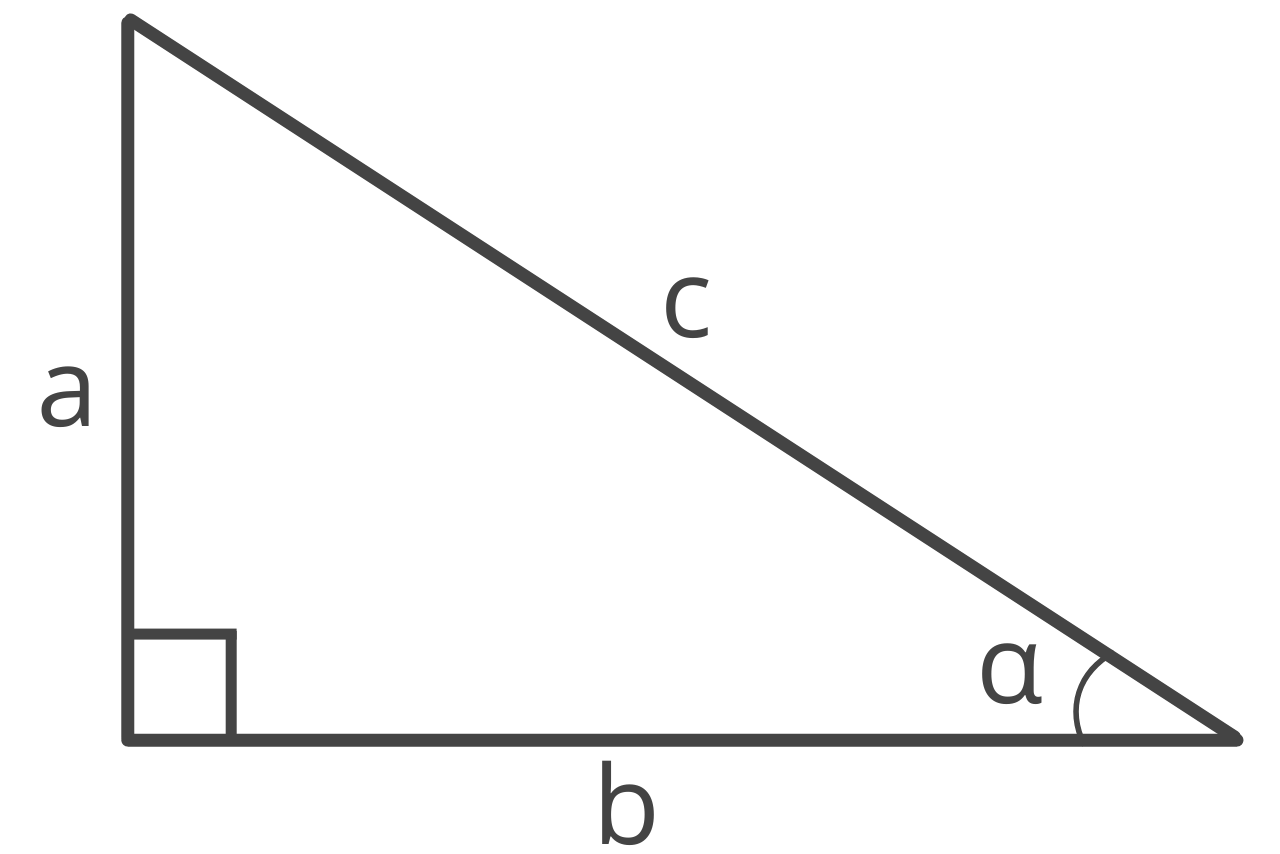# Secant Calculator – Calculate sec(x)

Find the secant of an angle using the sec calculator below. Start by entering the angle in degrees or radians.

## How to Find the Secant of an Angle

In a right triangle, the secant of angle α, or sec(α), is the ratio between the adjacent side of an angle and the hypotenuse.Secant is a trigonometric function abbreviated sec. Use the formula below to calculate the secant of an angle.

### Secant Formula

The secant formula is:

sec(α) = hypotenuse / adjacent = c / b

Thus, the secant of angle α in a right triangle is equal to the length of the hypotenuse c divided by the adjacent side b.

To find the ratio of sec, simply enter the length of the hypotenuse and adjacent side, then simplify.This formula might look very similar to the formula to calculate cosine. That’s because secant is the reciprocal of cosine.

For example, let’s calculate the secant of angle α in a triangle with the length of the hypotenuse equal to 8 and the adjacent side equal to 6.

sec(α) = 8 / 6 = 4 / 3

## Secant vs. Arccos

Secant should not be confused with arccos, which is the inverse of the cosine function. The difference being that secant is equal to 1/cos(x), while arccos is the inverse of the cosine function.

sec(x) = 1 / cos(x) = [cos(x)]-1

Whereas,

arccos(y) = x or cos-1(y) = x when y = cos(x)

## Secant Graph

If you graph the secant function for every possible angle, it forms a series of repeating U-curves.One important property to note in the graph above is that the secant of an angle is never in the range of -1 to 1; it’s always smaller than or equal to -1 or larger than or equal to 1.

You’ll also notice that the curves never cross the x-axis.

The secant function has asymptotes where the cosine function has x-intercepts. This is due to the reciprocal of 0 being undefined. The asymptotes occur every π radians, or 180°. See values in the table below.

The secant function continues indefinitely and has a period of 2π. The relative maximum and minimum values occur in each period and are separated by a distance of one period.

## Secant Table

The table below shows common angles and the sec value for each of them.

Table showing common angles and sec values for each.
0 1
15° π / 12 √6 – √2
30° π / 6 2√3 / 3
45° π / 4 √2
60° π / 3 2
75° / 12 √6 + √2
90° π / 2 undefined
105° / 12 -√6 – √2
120° / 3 -2
135° / 4 -√2
150° / 6 2√3 / 3
165° 11π / 12 -√6 – √2
180° π -1
195° 13π / 12 -√6 – √2
210° / 6 2√3 / 3
225° / 4 -√2
240° / 3 -2
255° 17π / 12 -√6 – √2
270° / 2 undefined
285° 19π / 12 √6 + √2
300° / 3 2
315° / 4 √2
330° 11π / 6 2√3 / 3
345° 23π / 12 √6 – √2
360° undefined

You might also be interested in our cosecant and cotangent calculators.

### Do you use degrees or radians with secant?

Although you can use either degrees or radians with secant, radians are the most common unit of measurement. This is due to the simplicity when calculating formulas that involve trigonometric functions in radians.

### Is the secant for a negative angle the same as a positive angle?

Yes, the identity for the secant of a negative angle is sec(-x) = sec(x) for angles that have the same reference angle.

### Can you find a secant without a calculator?

To find the secant of an angle without a calculator, you need to know the unit circle and the trigonometric ratios.

First, identify the reference angle for the given angle.

Then, determine the cosine of the reference angle, and use the reciprocal of this value to find the secant of the original angle.

### How do you find secant on a calculator?

To find the secant of an angle with a calculator, begin by entering the angle in degrees or radians (depending on what mod you calculator is in). Press the cos button on the calculator and then take the reciprocal of the cosine value to find secant.